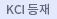#검색
다국어 입력

http://chineseinput.net/에서 pinyin(병음)방식으로 중국어를 변환할 수 있습니다.

변환된 중국어를 복사하여 사용하시면 됩니다.

예시)
• 中文 을 입력하시려면 zhongwen을 입력하시고 space를누르시면됩니다.
• 北京 을 입력하시려면 beijing을 입력하시고 space를 누르시면 됩니다.
닫기

검색결과 좁혀 보기

• 좁혀본 항목 보기순서

• 원문유무
• 음성지원유무
• 원문제공처
• 등재정보
• 학술지명
• 주제분류
• 발행연도
• 작성언어
• 저자

오늘 본 자료

• 오늘 본 자료가 없습니다.
더보기
• 무료
• 기관 내 무료
• 유료
•TSSG growth, morphology and properties of potassium lithium niobate (KLN) crystals

In the present paper, potassium lithium niobate(KLN) crystals have been grown along <001>, <100> and <110> directions by the top seeded solution growth (TSSG) method from Li-richer melts with different compositions. The morphologies of KLN crystals grown along different directions have been studied, and the well-developed facets have been unambiguously indexed using X-ray goniometer and stereographic projection analysis. The growth mechanism and defects such as cracks and inclusions were discussed on the basis of observations of facets on the crystal-solution interfaces. The crystal compositions were determined by a chemical analysis method. The structure and lattice constants of KLN crystals were determined and calculated on the basis of XRD data by using TREOR90 and PIRUM programs. The Curie temperature and optical absorption were determined by dielectric constant peak and spectrum measurements. respectively. The blue second harmonic generation (SHG) characteristics of KLN sample were also investigated using a pulsed dye laser.

•Degradation Model and Evaluation Criteria for the Seismic Behavior of Corrosion H-section Steel Column

The cross section would be reduced and the material properties of the steel structure would be deteriorated under the corrosion environment, which will have different effects on the earthquake resistant behavior. This paper takes corroded H-steel column as the research object. Firstly, the influence on the failure mode, bearing capacity, hysteretic properties, ductility and hysteretic energy is analyzed from specific factors which consist of different width-to-thickness on flange, different height-to-thickness on web and different axial compression ratios by FEA. The results show that, the area of the hysteresis loop diminishes gradually, the bearing capacity decrease clearly, the accumulative energy dissipation and ductility coefficient decreased continuously, and the degradation laws of buckling load, peak load, hysteretic energy and ductility coefficient of corroded H-steel columns are thereby established. When the thickness of flange decreased by 30%, the peak load decreased by 32% and hysteretic energy decreased by 77%. However, the peak load decreased by 14% and hysteretic energy decreased by 40% when the thickness of web decreased by 30%. When the axial compression ratio is 0.6, and the mass loss ratio of corroded H-steel columns reached 22%, the peak load and hysteretic energy decreased by 28% and 51% respectively. Secondly, the hysteretic model of load-displacement of corroded H-steel members under cyclic loading is proposed based on the simulation results and the degradation model of characteristic point of skeleton line was given. Finally, the evaluation criteria are proposed based on the seismic performance objectives, and the classification of failure types as well as the overall performance evaluation of corroded steel members are carried out.

•• LOCAL APPROXIMATE SOLUTIONS OF A CLASS OF NONLINEAR DIFFUSION POPULATION MODELS

This paper studies approximate solutions for a class of nonlinear diffusion population models. Our methods are to use the fundamental solution of heat equations to construct integral forms of the models and the well-known Banach compression map theorem to prove the existence of positive solutions of integral equations. Non-steady-state local approximate solutions for suitable harvest functions are obtained by utilizing the approximation theorem of multivariate continuous functions.

••ON THE EQUATION f"'+ff"+$\lambda(l-f'^2)=0$ WITH $\lambda\leq-\frac{1}{2}$ ARISING IN BOUNDARY LAYER THEORY

For any fixed $\lambda\leq-\frac{1}{2}$, there exists $f(\eta){\in}C^1[0,+\infty)$ which satisfies the following nonlinear boundary value problem f'+ff'+$\lambda(l-f'^2)=0$ a.e.in $(0,+\infty)$, f(0)=0, f'(0) = 0, $f'(+\infty)=1$, which arises in boundary layer theory in fluid mechanics.

•THE GENERALIZED INVERSE ${A_{T,*}}^{(2)}$ AND ITS APPLICATIONS

The existence and representations of some generalized inverses, including ${A_{T,*}}^{(2)},\;{A_{T,*}}^{(1,2)},\;{A_{T,*}}^{(2,3)},\;{A_{*,S}}^{(2)},\;{A_{*,S}}^{(1,2)}\;and\;{A_{*,S}}^{(2,4)}$, are showed. As applications, the perturbation theory for the generalized inverse {A_{T,S}}^{(2)} and the perturbation bound for unique solution of the general restricted system $A_{x}$ = b(dim(AT)=dimT, $b{\in}AT$ and $x{\in}T$) are studied. Moreover, a characterization and representation of the generalized inverse ${A_{T,*}}^{(2)}$ is obtained.

•Effect of single-pulse laser irradiation energy on healing fatigue damage for copper film

In this study, the optimum laser process parameter was determined by fatigue experiment for the treated copper film specimens withthickness of 25 μm. The mechanism of healing fatigue damage was analyzed by the numerical simulation of temperature field induced bylaser irradiation and the microstructure changes on the surface of specimens. The results showed that the fatigue damage of copper filmspecimens can be healed when the applied energy density is in the range from 4×10³J/m² to14×10³ J/m², and the fatigue life can increaseby about 5 times when the applied energy density is 7×10³ J/m². The process hardening and grain refinement in the surface layer aremainly responsible for extending fatigue life.